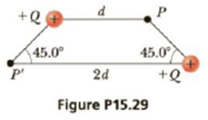Chapter 15, Problem 29P

Chapter
Section
Textbook Problem

Two equal positive charges are at opposite corners of a trapezoid as in Figure P15.29. Find symbolic expressions for the components of the electric field at the point P.To determine
The particle’s acceleration.

Explanation

Given info: The charge of the particle is 3.00pC . The mass of the particle is 1.00×109kg . Electric field strength is 2.00×103N/C .

The free body diagram is,

From the figure,

x=(d2)cos45ο=d2

The x component of electric field is given by,

Ex=E'Ecos45ο

• E is the electric field due to the bottom charge.
• E' is the electric field due to the charge at the top.

The y component of electric field is given by,

Ey=Esin45ο

Formula to calculate theelectric field due to the bottom charge is,

E=keQx2

Formula to calculate theelectric field due to the charge at the top is,

E'=keQd2

From the above equations,

Ex

Still sussing out bartleby?

Check out a sample textbook solution.

See a sample solution

The Solution to Your Study Problems

Bartleby provides explanations to thousands of textbook problems written by our experts, many with advanced degrees!

Get Started